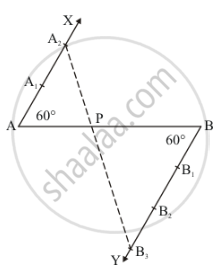Share

# Determine a Point Which Divides a Line Segment of Length 12 Cm Internally in the Ratio 2 : 3 Also, Justify Your Construction. - CBSE Class 10 - Mathematics

#### Question

Determine a point which divides a line segment of length 12 cm internally in the ratio 2 : 3 Also, justify your construction.

#### Solution

Given that

Determine a point which divides a line segment of length 12cminternally in the ratio of 2:3.

We follow the following steps to construct the givenStep of construction

Step: I- First of all we draw a line segment AB = 12 cm.

Step: II- We draw a ray AX making an acute angle ∠BAX = 60° with AB.

Step: III- Draw a ray BY parallel to AX by making an acute angle ∠ABY = ∠BAX.

Step IV- Mark of two points A1, A2 on AX and three points B1, B2, B3 on BY in such a way that AA1 = A1A2 = BB1 = B1B2 = B2B3.

Step: V- Joins A2B3 and this line intersects AB at a point P.

Thus, P is the point dividing AB internally in the ratio of 2:3

Justification:

In ΔAA2P and ΔBB3P we have

∠A2AP = ∠PBB3 [∠ABY = ∠BAX]

And ∠APA2 = ∠BPB3    [Vertically opposite angle]

So, AA similarity criterion, we have

ΔAA2P ≈ ΔBB3P

(A A_2)/(BB_3)=(AP)/(BP)

(AP)/(BP)=2/3

Is there an error in this question or solution?

#### APPEARS IN

Solution Determine a Point Which Divides a Line Segment of Length 12 Cm Internally in the Ratio 2 : 3 Also, Justify Your Construction. Concept: Division of a Line Segment.
S